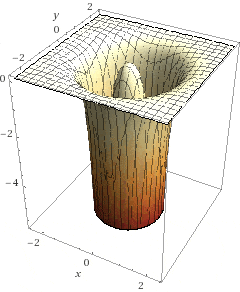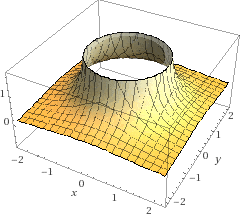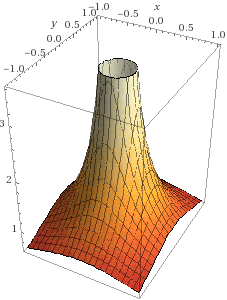# Electric Field Outside of a Ring of Charge in the X-Y Plane

• Zap
In summary, In Coulomb's law, the electric field in the x-y plane of a ring of charge resting at the origin on the x-y plane is zero. However, if the point of interest is placed on the x-axis, the electric field is radial and independent of the angle.f

#### Zap

If I try to calculate the electric field in the x-y plane of a ring of charge resting at the origin on the x-y plane, I keep getting zero.

$$dE(r) = \frac{1}{4\pi\epsilon}\frac{dq}{r^2} = \frac{\lambda R}{4\pi\epsilon}\frac{d\theta}{r^2},$$

in which ##\vec R## is a vector with magnitude equal to the radius of the disk that points from the origin to an infinitesimal charge ##dq##, and ##d\theta## is an angle of infinitesimal displacement that the vector ##\vec R## makes relative to the horizontal.

Then, I use the Law of Cosines to define ##r^2## in terms of ##\theta##,

$$r^2 = R^2 + P^2 - 2RPcos(\theta - \phi),$$

in which ##\vec P## is a position vector that points from the origin to the point of interest with magnitude ##P=\sqrt{x^2 + y^2}##, ##\theta## is the angle ##\vec R## makes with the horizontal, ##\phi## is the angle ##\vec P## makes with the horizontal and ##\vec r## is the distance from the infinitesimal charge ##dq## to the point of of interest, so that ## \vec P - \vec R = \vec r ##.

Using the identity ## cos(\theta - \phi) = cos \theta cos \phi + sin \theta sin \phi ## and ##tan\phi = \frac{y}{x}##,

$$r^2 = R^2 + P^2 - 2R(xcos\theta + ycos\theta),$$

$$dE(P) = \frac{\lambda R}{4\pi\epsilon}\frac{d\theta}{R^2 + P^2 - 2R(xcos\theta + ycos\theta)},$$

$$dE_x(P) = dE(P)cos\phi =\frac{\lambda R}{4\pi\epsilon} \frac{x}{P} \frac{d\theta}{R^2 + P^2 - 2R(xcos\theta + ycos\theta)}$$

and

$$E_x(P) = dEcos\phi =\frac{\lambda R}{4\pi\epsilon} \frac{x}{P} \int \frac{d\theta}{R^2 + P^2 - 2R(xcos\theta + ycos\theta)} = 0$$ from ##\theta = 0 ## to ##2\pi##.

How is it possible?

•Delta2
I assume that the point of interest is outside the circle in the xy-plane, correct?
If I were you, I would make the solution less complicated by observing that, without loss of generality, you can pick the point of interest on the x-axis. By symmetry the field must be radial and independent of the angle, so you might as well set ##y=0## and ##P=x##. This will simplify the integral which is not zero, by the way.

•Delta2
I’ve no idea how to actually solve that integral, but accordng t Wolfram Alpha, it is zero. Is the integral correctly set up? Perhaps, Wolfram Alpha is incorrect.

I’m aware of the solution along the axis. I guess I thought that a more general solution would be different, but as you have pointed out, it should be independet of the angle. So, I should be able to just swap x or y with P after solving the integral along the axis.

Thanks.

Last edited:
•Delta2
I’ve no idea how to actually solve that integral, but accordng t Wolfram Alpha, it is zero. Is the integral correctly set up? Perhaps, Wolfram Alpha is incorrect.
If you are wondering if an integral is zero, it is a good idea to plot the integrand and look at the area under the curve. In this case you don't even have to do that. In the simplified form, the integrand is$$\frac{1}{R^2-2xR\cos \theta+x^2}$$ The minimum value of the denominator is ##(R-x)^2## and the maximum value is ##(R+x)^2##. This means that the integrand is always positive, therefore the integral must be positive and never zero.

Thanks for the tip!

I just noticed that there is another problem with your setup. You say that ##dE_x(P)=dE(P)\cos \phi## with ##\cos \phi =x/R##. This is not correct. Draw a good drawing and you will see why. It is best to start with $$d\vec E=\frac{1}{4\pi \epsilon_0}\frac{\lambda R~d\theta~(\vec r-\vec{r}')}{| \vec r-\vec{r}'|^3}$$then substitute ##\vec r=x~\hat x##, ##\vec {r}'=R\cos \theta ~\hat x+R\sin \theta ~\hat y## and pick out the x-component.

•Delta2
The integral still looks pretty ugly after setting y to zero.

I got

$$\int \frac{(x-Rcos\theta) d \theta}{(R^2 + x^2 - 2Rxcos\theta)^2},$$

which Wolfram still says is zero over the interval.

I confused this result with the one on the axis of the ring, which is well known. This is on the plane of the ring, and I can't find any solution for it on the web, except for a complicated approximation:

http://student.ndhu.edu.tw/~d9914102/Teaching/EM/Paper/data/Off-axis electric field of a ring of charge_Project Paper.pdf

I went ahead and cleaned up their result with my own terms and set ##\theta = \frac{1}{2}\pi## for ##E_x## and got

$$E(P) = \frac{Q}{4 \pi \epsilon} \frac{P}{\pi} \frac{\pi (R^2 + P^2) - 2RP(\pi - \sqrt{2}) - 2\sqrt{2}R^2}{(R^2 + P^2)^\frac{3}{2} (R - P)^2}.$$

Or, for the sake of cleanliness,

$$E(P) ≈ \frac{Q}{4 \pi} \frac{P^2(P-2R)}{(R^2 + P^2)^\frac{3}{2} (R - P)^2} ,$$

if we let ##\frac {\sqrt{2}}{\pi} ≈ \frac{1}{2}## and ##\epsilon = 1## for atmosphere.

So, if we let ##R → 0##,

$$E(P) → \frac{Q}{4\pi} \frac{1}{P^2}.$$

Finally, a 3D plot looks good, with ##R## and ##\frac{Q}{4\pi} = 1##.However, the electric field seems to die off really fast.

I am now wondering if I can take this result and create a cylindrical shell of charge.

#### Attachments

Last edited:
Sorry. Apparently the approximation ##\frac{\sqrt{2}}{\pi} = \frac{1}{2}## fails near the radius of the ring. That's why the electric field becomes super negative on the 3D plot around the outer edge. I didn't think that tiny approximation would produce such a serious affect on the fields. The original solution presented in the paper seems to work very well, even though it's kind of ugly to look at ... I shouldn't have messed with it.

Here's what it should look like. All credit goes to the person in the paper, who is much smarter than I. Excellent work. I wish I could have solved it.#### Attachments

Last edited:
It’s actually a pretty bad model. It doesn’t seem to produce any realistic data at all. The fields are only somewhat similar to what they should be, but so would have been ##\frac{a}{r}##, so I'm not sure what the point of it is.

Here's a plot of ##\frac{1}{r}##, which is a "charged ring." I give up.#### Attachments

Last edited:
zap please explain how you get
r^2=R^2+P^2 - 2R(xcos(theta)+ycos(theta)).I think it is r^2=R^2+P^2 - 2R(xcos(theta)+ysin(theta))

I don't remember. This is such a funny problem. The electric field along the axis is so easy to calculate. You can use Coulomb's law. Then, you try it off the axis and it becomes impossible. There's no point in deriving some approximate formula for the electric field over all space. It would have been much easier just to use a numerical integration algorithm. Too bad I didn't know that last year. That would have been awesome for my research. This is exactly why physics students should learn programming. It would have gave me a very quick solution to this problem.

But, now I know.